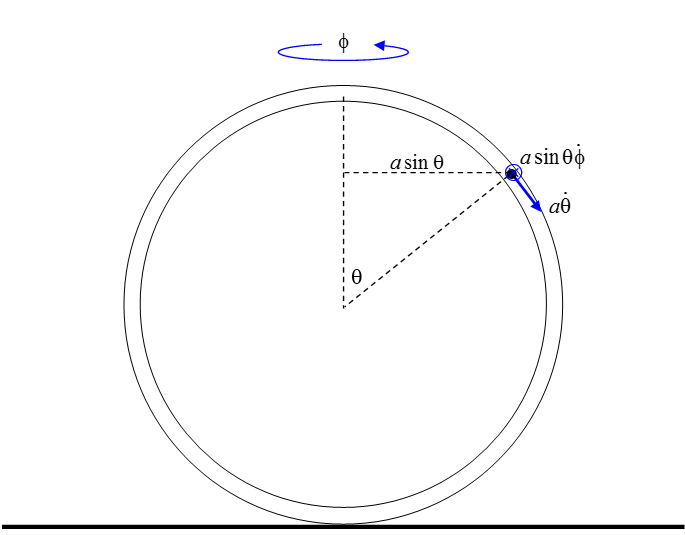$$\require{cancel}$$

13.8: More Lagrangian Mechanics Examples

•• Contributed by Jeremy Tatum
• Emeritus Professor (Physics & Astronomy) at University of Victoria

Example $$\PageIndex{1}$$The upper pulley is fixed in position. Both pulleys rotate freely without friction about their axles. Both pulleys are “light” in the sense that their rotational inertias are small and their rotation contributes negligibly to the kinetic energy of the system. The rims of the pulleys are rough, and the ropes do not slip on the pulleys. The gravitational acceleration is $$g$$. The mass $$M$$ moves upwards at a rate $$\dot{x}$$ with respect to the upper, fixed, pulley, and the smaller pulley moves downwards at the same rate. The mass $$m_{1}$$ moves upwards at a rate $$\dot{y}$$ with respect to the small pulley, and consequently its speed in laboratory space is $$\dot{x}-\dot{y}$$. The speed of the mass $$m_{2}$$ is therefore $$\dot{x}+\dot{y}$$ in laboratory space. The object is to find $$\ddot{x}$$ and $$\ddot{y}$$ in terms of $$g$$.

The kinetic energy is

$T=\frac{1}{2}M\dot{x}^{2}+\frac{1}{2}m_{1}(\dot{x}-\dot{y})^{2}+\frac{1}{2}m_{2}(\dot{x}+\dot{y})^{2}. \label{13.8.1}$

The potential energy is

$V=g[Mx-m_{1}(x-y)-m_{2}(x+y)]+constant. \label{13.8.2}$

Apply Lagrange’s equation (13.4.13) in turn to the coordinates $$x$$ and $$y$$:

$$x$$:

$M\ddot{x}+m_{1}(\ddot{x}+\ddot{y})+m_{2}(\ddot{x}+\ddot{y})=-g(M-m_{1}-m_{2}). \label{13.8.3}$

$$y$$:

$-m_{1}(\ddot{x}+\ddot{y})+m_{2}(\ddot{x}+\ddot{y})=-g(m_{1}-m_{2}) \label{13.8.4}$

These two equations can be solved at one’s leisure for $$\ddot{x}$$ and $$\ddot{y}$$.

Example $$\PageIndex{2}$$

A torus of mass $$M$$ and radius $$a$$ rolls without slipping on a horizontal plane. A pearl of mass $$m$$ slides smoothly around inside the torus. Describe the motion.I have marked in the several velocity vectors. The torus is rolling at angular speed $$\dot{\phi}$$ Consequently the linear speed of the centre of mass of the hoop is $$a\dot{\phi}$$ and the pearl also shares this velocity. In addition, the pearl is sliding relative to the torus at an angular speed $$\dot{\theta}$$ and consequently has a component to its velocity of $$a\dot{\theta}$$ tangential to the torus. We are now ready to start.

The kinetic energy of the torus is the sum of its translational and rotational kinetic energies:

$$\frac{1}{2}M(a\dot{\phi})+\frac{1}{2}(Ma^{2})\dot{\phi}^{2}=Ma^{2}\dot{\phi}^{2}$$

The kinetic energy of the pearl is

$$\frac{1}{2}ma^{2}(\dot{\theta}^{2}+\dot{\phi}^{2}-2\dot{\theta}\dot{\phi}\cos\theta)$$

Therefore

$T=Ma^{2}\dot{\phi}^{2}+\frac{1}{2}ma^{2}(\dot{\theta}^{2}+\dot{\phi}^{2}-2\dot{\theta}\dot{\phi}\cos\theta). \label{13.8.5}$

The potential energy is

$V=constant-mga\cos\theta. \label{13.8.6}$

The lagrangian equation in $$\theta$$ becomes

$a(\ddot{\theta}-\ddot{\phi}\cos\theta)+g\sin\theta=0. \label{13.8.7}$

The lagrangian equation in $$\phi$$ becomes

$(2M+m)\ddot{\phi}=m(\ddot{\theta}\cos\theta-\dot{\theta}^{2}\sin\theta) \label{13.8.8}$

These, then, are two differential equations in the two variables. The lagrangian part of the analysis is over; we now have to see if we can do anything with these equations.

It is easy to eliminate $$\ddot{\phi}$$ and hence get a single differential equation in $$\theta$$:.

$(2M+m\sin^{2}\theta)a\ddot{\theta}+ma\sin\theta\cos\theta\dot{\theta}^{2}+(2M+m)g\sin\theta=0. \label{13.8.9}$

If you are good at differential equations, you might be able to do something with this, and get $$\theta$$ as a function of the time. In the meantime, I think I can get the “first space integral” (see Chapter 6) – i.e. $$\dot{\theta}$$ as a function of $$\theta$$. Thus, the total energy is constant:

$Ma^{2}\dot{\phi}^{2}+\frac{1}{2}ma^{2}(\dot{\theta}^{2}+\dot{\phi}^{2}-2\dot{\theta}\dot{\phi}\cos\theta)-mga\cos\theta=E. \label{13.8.10}$

Equation $$\ref{13.8.8}$$ can easily be integrated once with respect to time, since $$\ddot{\theta}\cos\theta-\dot{\theta}^{2}\sin\theta=\frac{d}{dt}(\dot{\theta}\cos\theta)$$ as would have been apparent during the derivation of Equation $$\ref{13.8.8}$$. With the condition that the kinetic energy was initially zero, integration of Equation $$\ref{13.8.8}$$ gives

$(2M+m)\dot{\phi}=m\dot{\theta}\cos\theta. \label{13.8.11}$

Now we can easily eliminate $$\dot{\phi}$$ between Equations $$\ref{13.8.10}$$ and $$\ref{13.8.11}$$, to obtain a single equation relating $$\dot{\theta}$$ and $$\theta$$:

$b\dot{\theta}^{2}(1+c\sin^{2}\theta)-d\cos\theta-1=0, \label{13.8.12}$

where

$b=\frac{Mma^{2}}{(2M+m)E},\quad c=\frac{m}{2M},\quad d=\frac{mga}{E}=-\sec\alpha. \label{13.8.13 a,b,c}$

Example $$\PageIndex{3}$$As in example ii, we have a torus of radius $$a$$ and mass $$M$$, and a pearl of mass $$m$$ which can slide freely and without friction around the torus. This time, however, the torus is not rolling along the table, but is spinning about a vertical axis at an angular speed $$\dot{\phi}$$. The pearl has a velocity component $$a\dot{\theta}$$ because it is sliding around the torus, and a component $$a\sin\theta\dot{\phi}$$ because the torus is spinning. The resultant speed is the orthogonal sum of these. The kinetic energy of the system is the sum of the translational kinetic energy of the pearl and the rotational kinetic energy of the torus:

$T=\frac{1}{2}ma^{2}(\dot{\theta}^{2}+\sin^{2}\theta\dot{\phi}^{2})+\frac{1}{2}(\frac{1}{2}Ma^{2})\dot{\phi}^{2}. \label{13.8.14}$

If we refer potential energy to the centre of the torus:

$V=mga\cos\theta. \label{13.8.15}$

The lagrangian equations with respect to the two variables are:

$$\theta$$:

$a(\ddot{\theta}-\sin\theta\cos\theta\dot{\phi}^{2})-g\sin\theta=0. \label{13.8.16}$

$$\phi$$:

$m\sin^{2}\theta\dot{\phi}+\frac{1}{2}M\dot{\phi}=constant. \label{13.8.17}$

The constant is equal to whatever the initial value of the left hand side was. E.g., maybe the initial values of $$\theta$$ and $$\dot{\phi}$$ were $$\alpha$$ and $$\omega$$. This finishes the lagrangian part of the analysis. The rest is up to you. For example, it would be easy to eliminate $$\dot{\phi}$$ between these two equations to obtain a differential equation between $$\theta$$ and the time. If you then write $$\ddot{\theta}$$ as $$\dot{\theta}\frac{d\dot{\theta}}{d\theta}$$ in the usual way, I think it wouldn’t be too difficult to obtain the first space integral and hence get $$\dot{\theta}$$ as a function of $$\theta$$. I haven’t tried it, but I’m sure it’ll work.

Example $$\PageIndex{4}$$Figure XIII.10 shows a pendulum. The mass at the end is $$m$$. It is at the end not of the usual inflexible string, but of an elastic spring obeying Hooke’s law, of force constant $$k$$. The spring is sufficiently stiff at right angles to its length that it remains straight during the motion, and all the motion is restricted to a plane. The unstretched natural length of the spring is $$l$$, and, as shown, its extension is $$r$$. The spring itself is “light” in the sense that it does not contribute to the kinetic or potential energies. (You can give the spring a finite mass if you want to make the problem more difficult.) The kinetic and potential energies are

$T=\frac{1}{2}m(\dot{r}^{2}+(l+r)^{2}\dot{\theta}^{2}) \label{13.8.18}$

and

$V=constant-mg(l+r)\cos\theta+\frac{1}{2}kr^{2}. \label{13.8.19}$

Apply Lagrange’s equation in turn to $$r$$ and to $$\theta$$ and see where it leads you.

Example $$\PageIndex{5}$$

Another example suitable for lagrangian methods is given as problem number 11 in Appendix A of these notes.

Lagrangian methods are particularly applicable to vibrating systems, and examples of these will be discussed in Chapter 17. These chapters are being written in more or less random order as the spirit moves me, rather than in logical order, so that vibrating systems appear after the unlikely sequence of relativity and hydrostatics.